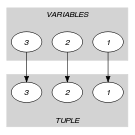## 5.418. vec_eq_tuple

Origin

Used for defining $\mathrm{𝚒𝚗}_\mathrm{𝚛𝚎𝚕𝚊𝚝𝚒𝚘𝚗}$.

Constraint

$\mathrm{𝚟𝚎𝚌}_\mathrm{𝚎𝚚}_\mathrm{𝚝𝚞𝚙𝚕𝚎}\left(\mathrm{𝚅𝙰𝚁𝙸𝙰𝙱𝙻𝙴𝚂},\mathrm{𝚃𝚄𝙿𝙻𝙴}\right)$

Arguments
 $\mathrm{𝚅𝙰𝚁𝙸𝙰𝙱𝙻𝙴𝚂}$ $\mathrm{𝚌𝚘𝚕𝚕𝚎𝚌𝚝𝚒𝚘𝚗}\left(\mathrm{𝚟𝚊𝚛}-\mathrm{𝚍𝚟𝚊𝚛}\right)$ $\mathrm{𝚃𝚄𝙿𝙻𝙴}$ $\mathrm{𝚌𝚘𝚕𝚕𝚎𝚌𝚝𝚒𝚘𝚗}\left(\mathrm{𝚟𝚊𝚕}-\mathrm{𝚒𝚗𝚝}\right)$
Restrictions
 $\mathrm{𝚛𝚎𝚚𝚞𝚒𝚛𝚎𝚍}$$\left(\mathrm{𝚅𝙰𝚁𝙸𝙰𝙱𝙻𝙴𝚂},\mathrm{𝚟𝚊𝚛}\right)$ $\mathrm{𝚛𝚎𝚚𝚞𝚒𝚛𝚎𝚍}$$\left(\mathrm{𝚃𝚄𝙿𝙻𝙴},\mathrm{𝚟𝚊𝚕}\right)$ $|\mathrm{𝚅𝙰𝚁𝙸𝙰𝙱𝙻𝙴𝚂}|=|\mathrm{𝚃𝚄𝙿𝙻𝙴}|$
Purpose

Enforce a vector of domain variables to be equal to a tuple of values.

Example
$\left(〈5,3,3〉,〈5,3,3〉\right)$

The $\mathrm{𝚟𝚎𝚌}_\mathrm{𝚎𝚚}_\mathrm{𝚝𝚞𝚙𝚕𝚎}$ constraint holds since the first, the second and the third items of $\mathrm{𝚅𝙰𝚁𝙸𝙰𝙱𝙻𝙴𝚂}=〈5,3,3〉$ are respectively equal to the first, the second and the third items of $\mathrm{𝚃𝚄𝙿𝙻𝙴}=〈5,3,3〉$.

Typical
 $|\mathrm{𝚅𝙰𝚁𝙸𝙰𝙱𝙻𝙴𝚂}|>1$ $\mathrm{𝚛𝚊𝚗𝚐𝚎}$$\left(\mathrm{𝚅𝙰𝚁𝙸𝙰𝙱𝙻𝙴𝚂}.\mathrm{𝚟𝚊𝚛}\right)>1$ $\mathrm{𝚛𝚊𝚗𝚐𝚎}$$\left(\mathrm{𝚃𝚄𝙿𝙻𝙴}.\mathrm{𝚟𝚊𝚕}\right)>1$
Symmetries
• Arguments are permutable w.r.t. permutation $\left(\mathrm{𝚅𝙰𝚁𝙸𝙰𝙱𝙻𝙴𝚂},\mathrm{𝚃𝚄𝙿𝙻𝙴}\right)$.

• Items of $\mathrm{𝚅𝙰𝚁𝙸𝙰𝙱𝙻𝙴𝚂}$ and $\mathrm{𝚃𝚄𝙿𝙻𝙴}$ are permutable (same permutation used).

Arg. properties

Contractible wrt. $\mathrm{𝚅𝙰𝚁𝙸𝙰𝙱𝙻𝙴𝚂}$ and $\mathrm{𝚃𝚄𝙿𝙻𝙴}$ (remove items from same position).

Used in

generalisation: $\mathrm{𝚕𝚎𝚡}_\mathrm{𝚎𝚚𝚞𝚊𝚕}$ ($\mathrm{𝚒𝚗𝚝𝚎𝚐𝚎𝚛}$ replaced by $\mathrm{𝚟𝚊𝚛𝚒𝚊𝚋𝚕𝚎}$ in second argument).

Keywords
Arc input(s)

$\mathrm{𝚅𝙰𝚁𝙸𝙰𝙱𝙻𝙴𝚂}$ $\mathrm{𝚃𝚄𝙿𝙻𝙴}$

Arc generator
$\mathrm{𝑃𝑅𝑂𝐷𝑈𝐶𝑇}$$\left(=\right)↦\mathrm{𝚌𝚘𝚕𝚕𝚎𝚌𝚝𝚒𝚘𝚗}\left(\mathrm{𝚟𝚊𝚛𝚒𝚊𝚋𝚕𝚎𝚜},\mathrm{𝚝𝚞𝚙𝚕𝚎}\right)$

Arc arity
Arc constraint(s)
$\mathrm{𝚟𝚊𝚛𝚒𝚊𝚋𝚕𝚎𝚜}.\mathrm{𝚟𝚊𝚛}=\mathrm{𝚝𝚞𝚙𝚕𝚎}.\mathrm{𝚟𝚊𝚕}$
Graph property(ies)
$\mathrm{𝐍𝐀𝐑𝐂}$$=|\mathrm{𝚅𝙰𝚁𝙸𝙰𝙱𝙻𝙴𝚂}|$

Graph model

Parts (A) and (B) of Figure 5.418.1 respectively show the initial and final graph associated with the Example slot. Since we use the $\mathrm{𝐍𝐀𝐑𝐂}$ graph property, the arcs of the final graph are stressed in bold.

##### Figure 5.418.1. Initial and final graph of the $\mathrm{𝚟𝚎𝚌}_\mathrm{𝚎𝚚}_\mathrm{𝚝𝚞𝚙𝚕𝚎}$ constraint(a) (b)
Signature

Since we use the arc generator $\mathrm{𝑃𝑅𝑂𝐷𝑈𝐶𝑇}\left(=\right)$ on the collections $\mathrm{𝚅𝙰𝚁𝙸𝙰𝙱𝙻𝙴𝚂}$ and $\mathrm{𝚃𝚄𝙿𝙻𝙴}$, and because of the restriction $|\mathrm{𝚅𝙰𝚁𝙸𝙰𝙱𝙻𝙴𝚂}|=|\mathrm{𝚃𝚄𝙿𝙻𝙴}|$, the maximum number of arcs of the final graph is equal to $|\mathrm{𝚅𝙰𝚁𝙸𝙰𝙱𝙻𝙴𝚂}|$. Therefore we can rewrite the graph property $\mathrm{𝐍𝐀𝐑𝐂}=|\mathrm{𝚅𝙰𝚁𝙸𝙰𝙱𝙻𝙴𝚂}|$ to $\mathrm{𝐍𝐀𝐑𝐂}\ge |\mathrm{𝚅𝙰𝚁𝙸𝙰𝙱𝙻𝙴𝚂}|$ and simplify $\underline{\overline{\mathrm{𝐍𝐀𝐑𝐂}}}$ to $\overline{\mathrm{𝐍𝐀𝐑𝐂}}$.